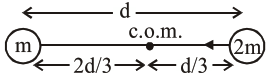Deepak Scored 45->99%ile with Bounce Back Crack Course. You can do it too!

# Two stars of masses

Question:

Two stars of masses $\mathrm{m}$ and $2 \mathrm{~m}$ at a distance $\mathrm{d}$ rotate about their common centre of mass in free space. The period of revolution is :

1. $\frac{1}{2 \pi} \sqrt{\frac{\mathrm{d}^{3}}{3 \mathrm{Gm}}}$

2. $2 \pi \sqrt{\frac{\mathrm{d}^{3}}{3 \mathrm{Gm}}}$

3. $\frac{1}{2 \pi} \sqrt{\frac{3 \mathrm{Gm}}{\mathrm{d}^{3}}}$

4. $2 \pi \sqrt{\frac{3 \mathrm{Gm}}{\mathrm{d}^{3}}}$

Correct Option: , 2

Solution:$\mathrm{F}=\frac{\mathrm{G}(2 \mathrm{~m}) \mathrm{m}}{\mathrm{d}^{2}}=(2 \mathrm{~m}) \omega^{2}(\mathrm{~d} / 3)$

$\frac{\mathrm{Gm}}{\mathrm{d}^{2}}=\omega^{2} \frac{\mathrm{d}}{3}$

$\Rightarrow \omega^{2}=\frac{3 \mathrm{Gm}}{\mathrm{d}^{3}}$

$\Rightarrow \omega=\sqrt{\frac{3 \mathrm{Gm}}{\mathrm{d}^{3}}}$

$\Rightarrow \mathrm{T}=\frac{2 \pi}{\omega}=2 \pi \sqrt{\frac{\mathrm{d}^{3}}{3 \mathrm{Gm}}}$